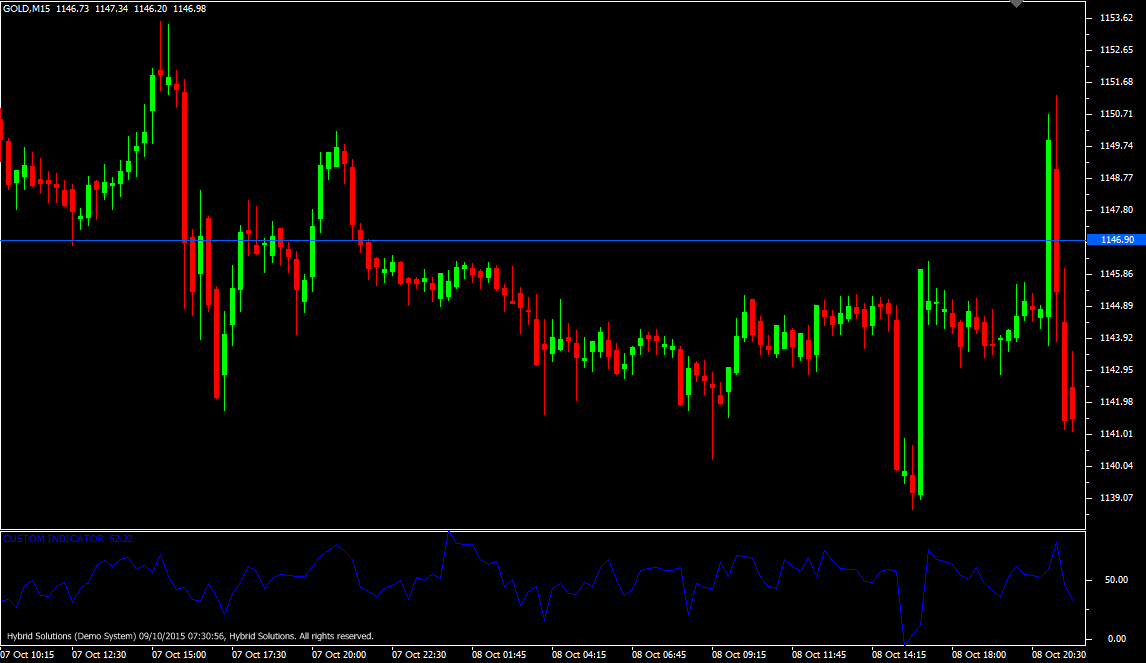# Strange Indicator

Free

Developed By: NeeduVerdeSolution

In stock

Strange Indicator is a VertexFX EMA(Exponential Moving Average) based indicator that calculates the direction of the trend and its strength.

At first we calculate the EMA of the Close, High and Low, and use the reference, SHIFT bars ago for our prices. Strange indicator is calculated by dividing the difference between the Close and Low of the EMA from the difference between High and Low of the EMA. This value is expressed as percentage (multiplied by 100).

SKU: 756 Categories: ,Strange Indicator is a client side VertexFX VTL EMA(Exponential Moving Average) based indicator that calculates the direction of the trend and its strength.

At first we calculate the EMA of the Close, High and Low, and use the reference, SHIFT bars ago for our prices. Strange indicator is calculated by dividing the difference between the Close and Low of the EMA from the difference between High and Low of the EMA. This value is expressed as percentage (multiplied by 100).

When the prices are moving downwards, and the markets starts stabilizing, the closing prices tend to be near the low of the candle, so the indicator exhibits lower values reflecting an end to the downtrend, whereas when the prices are moving upwards and start stabilizing, the closing prices tend to be farther from the low of the candles, so the indicator exhibits higher values – reflecting an end to the uptrend.

BUY – Place Buy position when indicator value closes above 30 from below.
SELL – Place Sell position when indicator value closes below 30 from above.